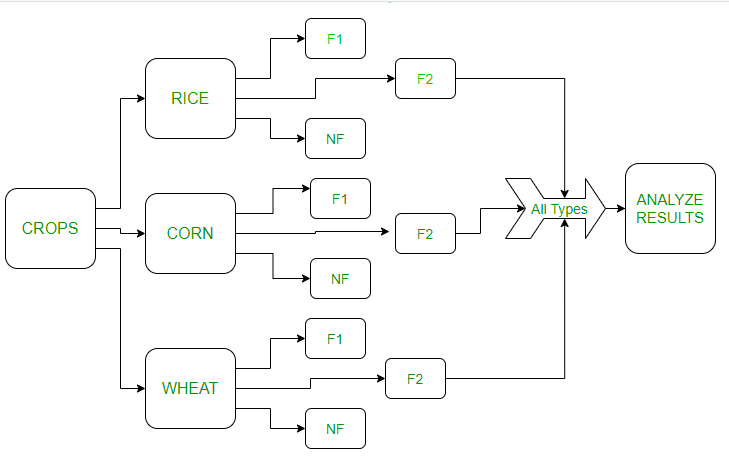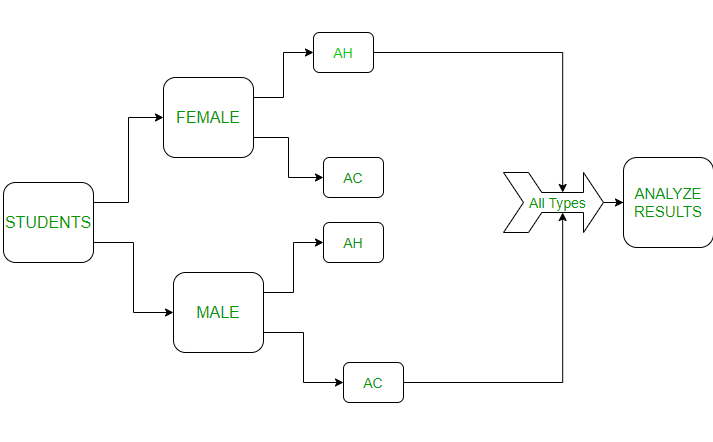Related Articles
Randomized Block Design with R Programming
• Last Updated : 22 Oct, 2020

Experimental Designs are part of ANOVA in statistics. They are predefined algorithms that help us in analyzing the differences among group means in an experimental unit. Randomized Block Design (RBD) or Randomized Complete Block Design is one part of the Anova types.

Randomized Block Design:

The three basic principles of designing an experiment are replication, blocking, and randomization. In this type of design, blocking is not a part of the algorithm. The samples of the experiment are random with replications are assigned to specific blocks for each experimental unit. Let’s consider some experiments below and implement the experiment in R programming.

### Experiment 1

Testing new fertilizers in different types of crops. Crops are divided into 3 different types(blocks). These blocks are again divided into 3 fertilizers that are used on those crops. The figure for this is as follows:In the above image:

F1 – Fertilizer 1, F2 – Fertilizer 2 , NF – No Fertilizer

The crops are divided into 3 blocks(rice, wheat, and corn). Then they are again divided into fertilizer types. The results of the different blocks will be analyzed. Let’s see the above in the R language.

Note: In R agricolae package can also be used for implementing RCBD. But here we are using a different approach.

Let’s build the dataframe:

## R

 `corn <- ``factor``(``rep``(``c``(``"corn"``, ``"rice"``, ``"wheat"``), each = 3)) ` `fert <- ``factor``(``rep``(``c``(``"f1"``, ``"f2"``, ``"nf"``), times = 3)) ` `corn`

Output:

``` corn  corn  corn  rice  rice  rice  wheat wheat wheat
Levels: corn rice wheat

```

## R

 `y <- ``c``(6, 5, 6,  ` `       ``4, 4.2, 5, ` `       ``5, 4.4, 5.5) ` ` `  `# y is the months the crops were healthy ` `results <- ``data.frame``(y, corn, fert) ` `  `  `fit <- ``aov``(y ~ fert+corn, data = results)                  ` `summary``(fit)`

Output:

```            Df Sum Sq Mean Sq  F value Pr(>F)
fert         2 1.4022  0.7011   6.505 0.0553 .
corn         2 2.4156  1.2078  11.206 0.0229 *
Residuals    4 0.4311  0.1078
---
Signif. codes:  0 ‘***’ 0.001 ‘**’ 0.01 ‘*’ 0.05 ‘.’ 0.1 ‘ ’ 1

```

Explanation:

The value of Mean Sq shows is blocking really necessary for the experiment. Here Mean Sq 0.1078<<0.7011 thus blocking is necessary will give precise values for the experiment. Though this method is a little debatable yet useful. The significance value of every experiment is given by the person taking the experiment. Here lets consider significance has 5% i.e 0.05. The Pr(>F) value is 0.553>0.05. Thus the hypothesis is accepted for the crops experiment. Let’s consider one more experiment.

### Experiment 2

Comparing the performances of students (male and female) blocks in different environments (at home and at college). To represent this experiment in the figure will be as follows:

Note: It generally is safe to consider an equal number of blocks and treatments for better results.In the above image:

AC – At College, AH: At Home

Students are divided into blocks as male and female. Then each block is divided into 2 different environments (home and college). Let’s see this in code:

## R

 `stud <- ``factor``(``rep``(``c``(``"male"``, ``"female"``), each = 2)) ` `perf <- ``factor``(``rep``(``c``(``"ah"``, ``"ac"` `), times = 2)) ` `perf`

Output:

``` ah ac ah ac
Levels: ac ah

```

## R

 `y <- ``c``(5.5, 5, ` `       ``4, 6.2) ` ` `  `# y is the hours students  ` `# studied in specific places ` `results <- ``data.frame``(y, stud, perf) ` `  `  `fit <- ``aov``(y ~ perf+stud, data = results)                  ` `summary``(fit)`

Output:

```Df Sum Sq Mean Sq F value Pr(>F)
perf         1 0.7225  0.7225   0.396  0.642
stud         1 0.0225  0.0225   0.012  0.930
Residuals    1 1.8225  1.8225

```

Explanation:

The value of Mean Sq is 0.7225<<1.8225,i.e, here blocking wasn’t necessary. And as Pr value is  0.642 > 0.05 (5% significance) and the hypothesis is accepted.

My Personal Notes arrow_drop_up
Recommended Articles
Page :